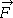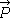# Forces on a Block and Friction on a Surface

JessicaHelena

## Homework Statement

A 2.5 kg block is initially at rest on a horizontal surface. A horizontal forceof magnitude 5.8 N and a vertical forceare then applied to the block. The coefficients of friction for the block and surface are μs = 0.43 and μk = 0.24.
(a) Determine the magnitude of the frictional force acting on the block if the magnitude ofis 8.0 N.

## Homework Equations

F_friction = Mu*F_normal

## The Attempt at a Solution

I really need help with this one, because I don't understand why I get a wrong answer.
Firstly, the net force in the y direction is 0. So F_g = P + F_N.
Then F_N = F_g - P = 2.5 * 9.8 - 8 = 16.5 N
Then since (I believe) the block is stationary, we use μs = 0.43, and so F_f = 0.43 * 16.5 =7.095.
Satisfied with my answer, I put this in the webassign, but it won't accept this as the correct answer. Where did I go wrong?

#### Attachments

Last edited by a moderator:

## Answers and Replies

Homework Helper
Gold Member
The formula ##f_s = \mu_s N## gives the maximum possible value for the static friction force. (It would be better to write the formula as ##f_s^{\rm max} = \mu_s N##.)

The static friction force in your situation need not be at its maximum possible value.

•Merlin3189
JessicaHelena
@TSny — so does that mean the WebAssign's looking for an answer that's like say, 7, rather than 7.095?

update: no, what I said above isn't right bc I checked... but how else should I interpret your hint?

Homework Helper
Gold Member
It would be better to write the formula as ##f_s^{\rm max} = \mu_s N##.
The form I use is ##|F_s|≤\mu_s|N|##. This emphasises that the direction of the frictional force is not dictated by the equation.

•TSny
JessicaHelena
@haruspex @TSny —Oh so since the maximum frictional force is 7.095N, but the other horizontal force being applied is 5.8 N, would the answer be 5.8 N?

Homework Helper
Gold Member
how else should I interpret your hint?
The relevant equation you quoted is incorrect. See either of the forms in posts 2 and 4.
Consider this: if the applied horizontal force is 5.8N and the frictional force in the other direction is 7N, what will happen to the block?

Homework Helper
Gold Member
@haruspex @TSny —Oh so since the maximum frictional force is 7.095N, but the other horizontal force being applied is 5.8 N, would the answer be 5.8 N?
Yes.

JessicaHelena
@haruspex @TSny — thank you! Can I ask another question though?

(c) Determine the magnitude of the frictional force acting on the block if the magnitude ofis 12.0 N.

So now that yields a maximum friction of 0.43*(2.5*9.8 - 12) = 5.375 N, which is less than the horizontal force F=5.8N. Then it's going to move right off the bat, right? How should I answer this?